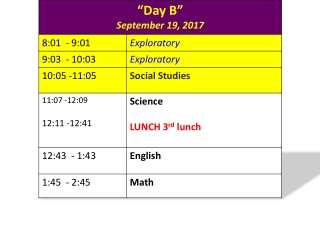DownloadDownload PresentationTake out your H.W., packet and notebook

# Take out your H.W., packet and notebook

Download Presentation## Take out your H.W., packet and notebook

- - - - - - - - - - - - - - - - - - - - - - - - - - - E N D - - - - - - - - - - - - - - - - - - - - - - - - - - -
##### Presentation Transcript

1. Take out your H.W., packet and notebook • What are some things that you notice? What are some things that you wonder.

2. Objective: • I will use estimationand place value to determine the placement of the decimal point in products • So I can determine how the size of the product is connected to each factor. • I will demonstrate my understanding when I can correctly write/place the decimal point in the product of a real world problem with my partners with at least 80% accuracy. 6.NS.B.3

3. Language Objective • By the end of the lesson, students will be able to use the language domains of reading, writing, listening and speaking to determine the product of multi-digit decimals. • Through the discussion, they will be able to orally use the math words like products, decimals and place value to show their understanding of how to determine the placement of the decimal point in the product. • “Math language” Vocabulary • product, place value, decimal, decimal point

4. Group work • Discussion • Classwork • Share Out • H.W/T.T.G

5. Page 46 Students work in small groups to complete the two given problems On the poster paper, students include > all work that supports solutions and the placement of the decimal in the answer. > groups will present their solutions and explain their supporting work > since all groups are presenting, each group present only one method of proving where the decimal should be placed.

6. Discussion (Page 46) Do you see a connection between the number of decimal digits in the factors and the product? In the first problem, there are two decimal digits in the first factor and one decimal digit in the second factor, which is a total of three decimal digits. The product has three decimal digits. a. 34. 62 × 𝟏2. 8 In the second problem, both factors have one decimal digit for a total of two decimal digits in the factors. The product also has two decimal digits. b. 𝟏𝟏. 𝟓 × 𝟏𝟑. 𝟓

7. Discussion (Page 46)

8. How do you feel? topic.

9. Page 49 #3 Krego earns \$2456.75 every month. He also earns an extra \$4.75 every time he sells a new gym membership. Last month, Krego sold 32 new gym memberships. How much money did Krego earn last month? (4.75 x 32) + 2456.75 =152 + 2456.75 =\$2608.75

10. CLASSWORK: pg.49 • Individually (8 minutes) work on page 49 • Work with a partner (10 minutes)

11. CLASSWORK: pg.49 1. 2. 3. 4.

12. Pgs. 47 and 48 Ticket to Leave . 4.9 x 1.001 = 4 9 0 4 9 Put where the decimal point will be in the answer.

13. Accommodations • Read or reread presentation or activity directions, as needed or after prompting • Use examples to model and act as a guide for emerging learners